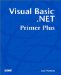# Review Questions

 1: What is a subroutine and why are they used in programs? A1: A subroutine is a series of program statements designed to accomplish a specific task. The advantage of a subroutine is that it can be called from any point in a program, thus reducing the need for duplicate code. This also reduces the amount of code that needs to be written, tested , debugged , and maintained . 2: What does the phrase "calling a subroutine" mean? A2: Calling a subroutine means that the program flow is about to be transferred to the code that comprises the subroutine. After all the statements in the subroutine body are executed, program flow is returned to the point following the subroutine call. The point at which the subroutine is called is often referred to as the caller. The caller is not a person per se. Rather, the caller refers to a point in the program where the subroutine is called. 3: What is a subroutine parameter? A3: Often, a subroutine needs some form of data to perform its task. This data is passed to the subroutine in the form of a parameter. For example, if a subroutine is supposed to calculate the amount of sales tax that is due from a sale, the subroutine might be called with parameters for the purchase price of the item, the sales tax rate, and the amount of sales tax. The last parameter would be empty when the parameter is passed to the subroutine and then filled in with the proper value when the subroutine has finished its task. 4: What is a symbolic constant? A4: A symbolic constant is simply a program value to which you have assigned a name . For example, you might use the statement: ` Const SalesTaxRate as Double = .06 ` You can then use the constant in an expression like: ` SalesTaxDue = PurchasePrice * SalesTaxRate ` Using symbolic constants helps to document how a statement is using the constant. 5: What is a stack? A5: A stack is a section of memory that can be used for a variety of programming tasks . With respect to subroutines, the stack is used to pass parameters and the return address to a subroutine. The stack behaves like a LIFO buffer. Data is pushed on the stack just before the subroutine is called, and then the data is popped off the stack in the subroutine. The subroutine uses the data that was popped off the stack for whatever purposes that data serves in the subroutine. The stack also contains the return address of where program execution is to resume once the subroutine finishes its task. 6: Why is a function different from a subroutine? A6: Functions and subroutines are very similar with one major exception: a function can return a value to the caller. The stack is used to return the value to the caller. 7: Write a function that converts a Fahrenheit temperature to Celsius. The formula is C = 5/9 (F “ 32) A7: The code for the program is ` Public Class frmTemperature Inherits System.Windows.Forms.Form ' Windows Form Designer generated code Private Sub btnCalc_Click(ByVal sender As System.Object, _ ByVal e As System.EventArgs) Handles btnCalc.Click txtCelsius.Text = Format(ConvertTemp(CDbl(txtFahrenheit.Text)),_ "###.00") End Sub Private Function ConvertTemp(ByVal Fahrenheit As Double) As Double Return ((5 / 9) * (Fahrenheit - 32)) End Function Private Sub btnExit_Click(ByVal sender As System.Object, _ ByVal e As System.EventArgs) Handles btnExit.Click Me.Dispose() End Sub End Class `Visual Basic .NET Primer Plus
ISBN: 0672324857
EAN: 2147483647
Year: 2003
Pages: 238
Authors: Jack Purdum

Similar book on Amazon